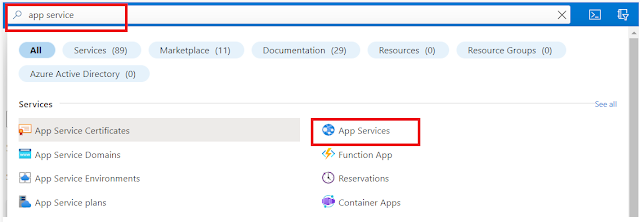### Basics of Python• Python was created by Guido van Rossum in 1991 as a general-purpose programming language.
• It is interpreted and interactive language.
• Python is an open-source language under a general public license (GPL).
• It supports both object-oriented and structured styles of programming.

Features:
• Cross-platform compatible: Python code can be executed on all platforms like Windows, Unix, Linux, and others.
• Open-source language.
• Supports both structured and object-oriented styles of programming.
• wide range of libraries.
• Provides interface required to connect all major databases like MySQL, Oracle, and Others
Knowledge of the below basic concepts is a must before proceeding with the coding.
• Identifiers
• Keywords
• variables
• datatypes
• Input, output statements
• Operators
1) Identifiers:
• Identifiers are used to identify any variable, function, class, module, and object in python by using a name which is called an Identifier.
• Identifiers are case-sensitive.
• It can start with an uppercase or lowercase character or an underscore (_) followed by n number of underscores, letters, and digits.
Example:  Number_1 = 50
Here Number_1 is an identifier

2) Keywords:
• Keywords are reserved words in python.
• These can't be used as an identifier to name a variable or function.
• Some commonly used keywords are given below
• if, elif, else, for, where, break, pass, continue, input
3) Variables:
• Variables are used to hold the data which are like a container.
• The value which the variable holds may not be the same throughout the program, it may change depending on the program logic.
• Syntax: Variable_name = value
Example: Number_1 = 50

In the above example, Number_1 is a container(variable) that holds the value 50

4) Datatypes:
• Every data belongs to some types. Commonly used data types are listed below
 Category Datatype Example Numeric int 450 long 32321343535675687 Numeric with a decimal point float 345.67 double 12453.5679873453 Alphanumeric char A string tutorials Boolean boolean True, False

1) Dynamical language - Python:

Example: Number_1 = 50
• In the above example, we didn't mention the data type at the time of declaring a variable but the python decides automatically based on the value which is assigned to it. This is called dynamic typing.
• Examples:
• Number_1 = 50  ---> Integer data type
• Number_1 = 'ÁBC'  ---> String data type
• Try this CODE:

5.1) Input statement:
• input() is a built-in function that is used to read input from the user using a standard input device (i.e., keyboard)
• It always returns string data irrespective of what it receives from the user.
• Syntax: Variable_name = input('Interactive Statement')
• Example:
• input_num = input("Enter the number:")
5.2) Output statement:
• print() is a built-in function which is used to print the output on a standard output device (i.e., monitor)
• Syntax: print(Variable_name)
• Examples:
• print(Number_1)
• print("Hello")
6) Operators:
• Operators are the symbols used to perform some operations on the program.
• The most commonly used operators are listed below
 Category Operators Arithmetic Operators +,-,*,/, %,// Relational Operators ==,!=,>,<,>=,<= Assignment Operators =,+=,-=,*=,/=,%= Logical Operators and, or, not

• These are the lines that are skipped during the execution of the program.
• There are two types of comments in python.
• Single line comment: starts with the # symbol and extends till the end of the line.
• Multi-line comment: starts with '' / ''' ends with ''/'''.
• Mainly used for document purpose
• Example:
• Single line comment: #program to demonstrate the basics of python
• Multi-line comment: ''''used for Demonstrating comment.''''
Let's see the basic concepts in detail in the next tutorial.